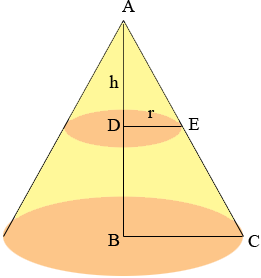SEARCH HOMEMath Central Quandaries & QueriesQuestion from Tammy: What is the ratio of the volume of the top half of a cone vs. the bottom half of the same cone? Is it seven times larger, or four times larger? and is the ratio consistent regardless of the size of the cone opening?Hi Tammy,

The volume of a cone is 1/3 π r2 h where r is the radius of the base and h is the height. In my diagram I let r and h be the radius and height of the upper half of the cone. Thus the height of the entire cone, the length of AB, is 2h.Triangles ABC and ADE are similar so what is the radius of the entire cone, the length of BC?

The volume of the upper half of the cone is 1/3 π r2 h. What is the volume of the entire cone? Subtract these to find the volume of the lower half of the cone. How does this value compare to the volume of the upper half?

PennyMath Central is supported by the University of Regina and The Pacific Institute for the Mathematical Sciences.